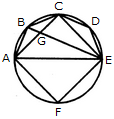# Non Verbal Reasoning - Analytical Reasoning

Exercise : Analytical Reasoning - Section 1
16.

Find the number of triangles in the given figure.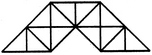23
27
29
31
Explanation:

The figure may be labelled as shown.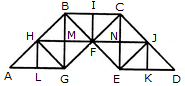The simplest triangles are AHL, LHG, GHM, HMB, GMF, BMF, BIF, CIF, FNC, CNJ, FNE, NEJ, EKJ and JKD i.e. 14 in number.

The triangles composed of two components each are AGH, BHG, HBF, BFG, HFG, BCF, CJF, CJE, JEF, CFE and JED i.e. 11 in number.

The triangles composed of four components each are ABG, CBG, BCE and CED i.e. 4 in number.

Total number of triangles in the given figure = 14 + 11 + 4 = 29.

17.

Find the number of triangles in the given figure.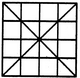36
40
44
48
Explanation:

The figure may be labelled as shown.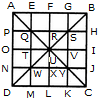The simplest triangles are APQ, AEQ, QTU, QRU, BGS, BHS, RSU, SUV, TUW, UWX, NWD, WDM, UVY, UXY, JCY and YKC i.e. 16 in number.

The triangles composed of two components each are QUW, QSU, SYU and UWY i.e. 4 in number.

The triangles composed of three components each are AOU, AFU, FBU, BIU, UIC, ULC, ULD and OUD i.e. 8 in number.

The triangles composed of four components each are QYW, QSW, QSY and SYW i.e. 4 in number.

The triangles composed of six components each are AUD, ABU, BUC and DUC i.e. 4 in number.

The triangles composed of seven components each are QMC, ANY, EBW, PSD, CQH, AGY, DSK and BJW i.e. 8 in number.

The triangles composed of twelve components each are ABD, ABC, BCD and ACD i.e. 4 in number.

Thus, there are 16 + 4 + 8 + 4 + 4 + 8 + 4 = 48 triangles in the figure.

18.

Find the number of triangles in the given figure.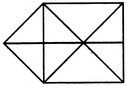15
16
17
18
Explanation:

The figure may be labelled as shown.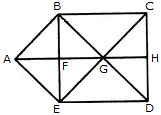The simplest triangles are ABF, BFG, BCG, CGH, GHD, GED, EFG and AFE i.e. 8 in number.

The triangles composed of two components each are ABG, BGE, AGE, ABE and GCD i.e. 5 in number.

The triangles composed of three components each are BCD, CDE, BED and BCE i.e. 4 in number.

Thus, there are 8 + 5 + 4 = 17 triangles in the figure.

19.

Find the number of triangles in the given figure.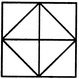8
10
12
14
Explanation:

The figure may be labelled as shown.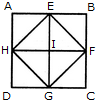The simplest triangles are AEH, EHI, EBF, EFI, FGC, IFG, DGH and HIG i.e. 8 in number.

The triangles composed of two components each are HEF, EFG, HFG and EFG i.e. 4 in number.

Thus, there are 8 + 4 = 12 triangles in the figure.

20.

Find the number of triangles in the given figure.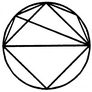8
10
11
12